# For the given slate of stress, determine the normal and shearing stresses exerted on the oblique lace of the shaded triangular element shown. Use a method of analysis based on the equilibrium of that element as was done in the derivations of Sec. 14.2.

Question-AnswerCategory: Strength of MaterialsFor the given slate of stress, determine the normal and shearing stresses exerted on the oblique lace of the shaded triangular element shown. Use a method of analysis based on the equilibrium of that element as was done in the derivations of Sec. 14.2.

For the given slate of stress, determine the normal and shearing stresses exerted on the oblique lace of the shaded triangular element shown. Use a method of analysis based on the equilibriumof that element as was done in the derivations of Sec. 14.2.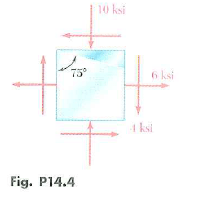Step: 1

Draw schematic diagram of the state of stress representing the normal and shear stress acting on the oblique face of the shaded triangular element.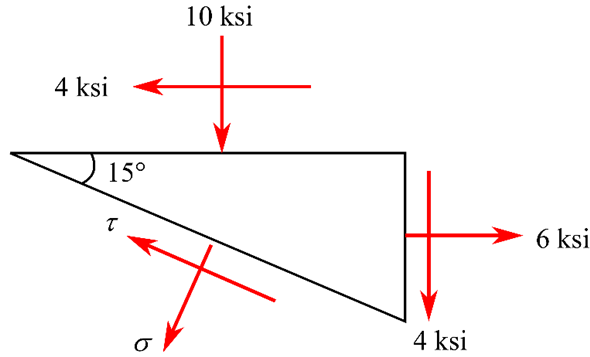Step: 2

Draw the schematic diagram showing the area of the oblique plane.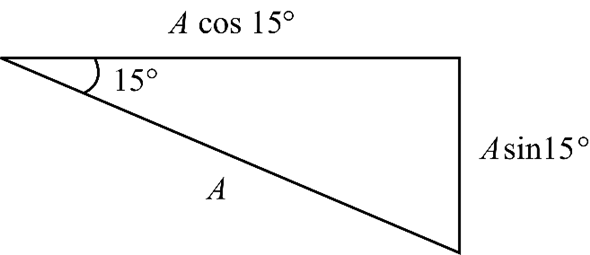Step: 3

Draw the free body diagram of the triangular stress element by multiplying the area normal to the stress.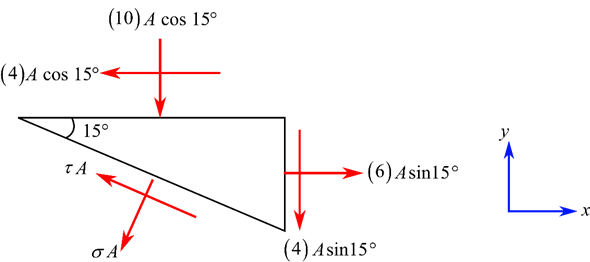Step: 4

Consider the algebraic sum of forces along the x-axis to be zero.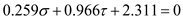…… (1)
Here, A is the area of the stress element,is the normal stress, and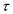is the shear stress.
Consider the algebraic sum of forces along the x-axis to be zero.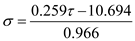…… (2)

Step: 5

Substitute equation (2) in (1).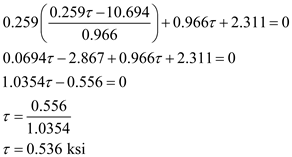Thus, the magnitude of shear stress on the oblique face is.

Step: 6

Substitute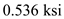forin equation (2).Here, “” indicates normal stress acts in opposite direction.
Thus, the magnitude of normal stress on the oblique face is.

Step: 7

Draw the triangular stress element representing the normal and shear stress.